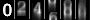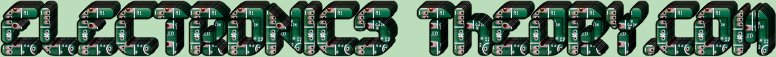# Other Types of Meters

The Voltmeter is very handy in electronics work. But there are times when other meters are needed to do the job. And many of these meters are also built upon the D'Arsonval movement. For instance, how would you measure the resistance of a resistor that is not in a circuit? This would require the use of an Ohmmeter.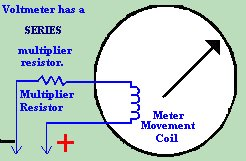Or what if you wanted to know the amount of current flowing through a circuit, so that you knew what size of fuse to put in the circuit? You would need an Ammeter to measure the current in Amps.

Recall that the Voltmeter had a resistor in series with it. This resistor, called the "Multiplier Resistor" was used to calibrate the meter to work within a given range. A Voltmeter is also placed in parallel with the circuit in test.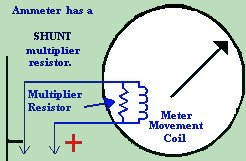An Ammeter, on the other hand, is built with a resistor in PARALLEL or in SHUNT with the D'Arsonval movement's coil. In the case of the Ammeter, the SHUNT resistor is of a very low resistance. Much lower resistance, in fact, than the coil in the meter movement. Remember finding resistance in a parallel circuit? The two resistors in parallel carry more current than either of the resistors by themselves. This is because the combined resistance is lower than the lowest resistor in the parallel network. Also, the resistor with the lowest resistance always carries the greatest current. This is of utmost importance here. If too much current were to go through our sensitive meter coil, it would burn up and destroy the coil, hence making the meter useless. The answer, of course, is to make sure that no matter HOW high the current, the majority of the current will always flow through the shunt resistor. It is for this reason that the shunt resistor has a lower resistance value than the coil winding of the meter itself. The formula we use for finding the value of the shunt resistor is as follows: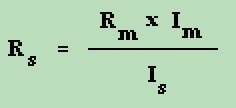Where: R s = shunt resistance
R m = meter movement resistance
I m = full scale meter movement current
I s = shunt current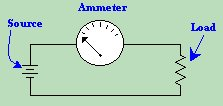The Ammeter, unlike the Voltmeter, is not used in parallel with the circuit in test. Rather, the ammeter is placed in series with the circuit - essentially becoming an integral part of the circuit in test. If a Voltmeter were to be removed from a circuit in test, the circuit would continue to run. If an Ammeter were to be removed while the circuit were running, the circuit would shut down, because there would no longer be continuity or flow of electricity.

Finally, the Ohmmeter is one of the most used tools on the electronics workbench. It is used not only to measure the resistance value of a given resistor or circuit component, but also to check continuity of wire, to test for opens and shorts in a circuit, and many other things. But an ohmmeter is not self sufficient.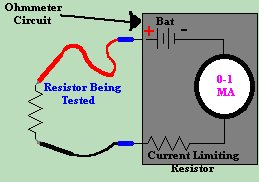The Ohmmeter is made up of an Ammeter, a battery, and a CURRENT LIMITING RESISTOR . As shown in the picture to the side, the battery causes a current to flow through the meter. We know the value of the current limiting resistor, so if we short the meter leads together, we know how much current the meter should indicate. If the meter indicates a lower current value than we expected, then there must be some added resistance. Therefore, we can use this device to detect, and to measure the value, of an outside resistance.

 (On The Following Indicator... PURPLE will indicate your current location) 1 2 3 4 5 6 7 8 9 10 11 12 13 14 15 16 17 18 19 20 21 22 23 24 25 26 27 28 29 30 31 32 33 34 35 36 37 38 39 40 41 42 43 44 45 46 47 48 49 50 51 52 53 54 55 56 57 58 59 60 61 62 63 64 65 66 67 68 69 70 71 72 73 74 75

[COURSE INDEX] [ELECTRONICS GLOSSARY] [HOME]Otherwise - please click to visit an advertiser so they know you saw their ad!

VISITORS: